Arduino

# Machine Learning for Makers: How to Control DC Motors With Voice Commands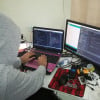June 29, 2018 by Muhammad Aqib
ShareUse the machine learning program Wekinator and an Arduino UNO to control DC Motors with your voice!

## Software

 1 Wekinator

## Hardware

 1 L298N 1 FSA8049UCX

In this article, part 4 of a larger machine learning series, you will learn about controlling DC motors using voice commands. We will be using the Wekinator software as a machine learning platform, and after training Wekinator, we will get the output using processing and send it to the Arduino.

Catch up on the rest of the Wekinator Machine Learning series here:

### Circuit Diagram

Make the connections as follows:

• ENA of L298N to pin 11 of Arduino
• ENB of L298N to pin 10 of Arduino
• IN1 of L298N to pin 9 of Arduino
• IN2 of L298N to pin 8 of Arduino
• IN3 of L298N to pin 7 of Arduino
• IN4 of L298N to pin 6 of Arduino
• Connect the positive of battery source you are using to the 12V connector of L298N, the negative of the battery to GND of L298N, and the GND of L298N to the GND of Arduino
• In the end, connect the two motors at the two ends of L298N### How to Run the Program

First of all, copy and paste the Arduino code at the end of this post in Arduino IDE and upload the code.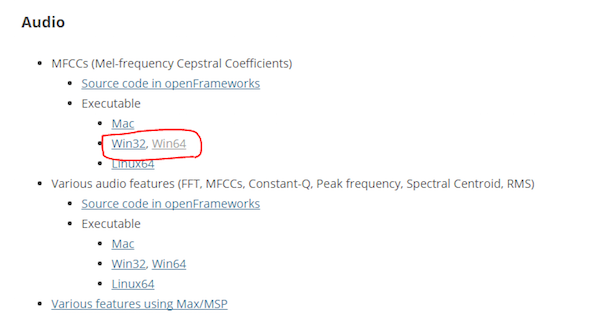After downloading, unzip it and run the “.exe” file. It will look like as shown below. Now you will need a microphone to give the input to Wekinator. If you have attached an external microphone, then make sure that it is selected in the sound settings.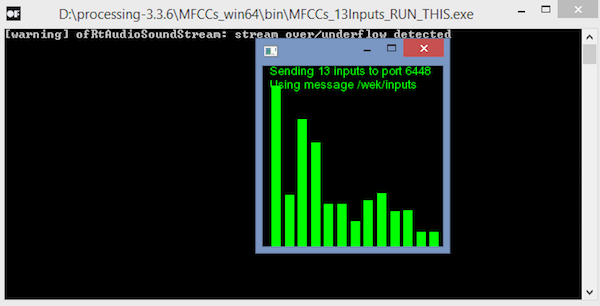You will need another sketch to get the output from Wekinator. This sketch is given at the end of this post. Paste it into the new processing window and run the sketch.

Open the Wekinator and make the settings as shown in the figure below. Set the inputs to 13 and the outputs to 1. Set the type to “All Dynamic time warping” with 5 gesture types and click on “next”.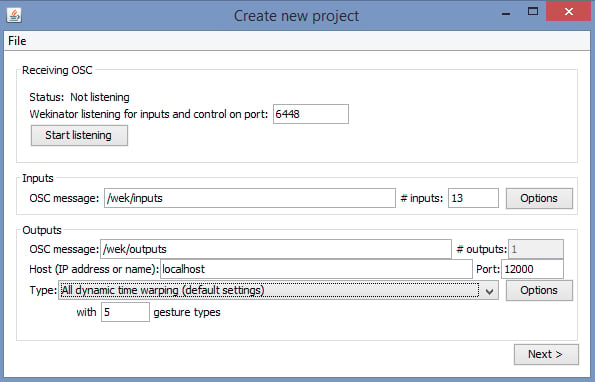Now hold the “+” button in front of output_1 and say “forward”.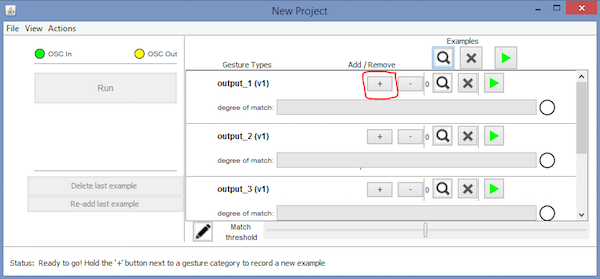Then hold the “+” button in front of output_2 and say “backward”.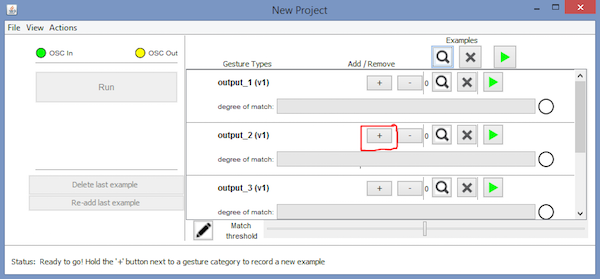Then hold the “+” button in front of output_3 and say “right”.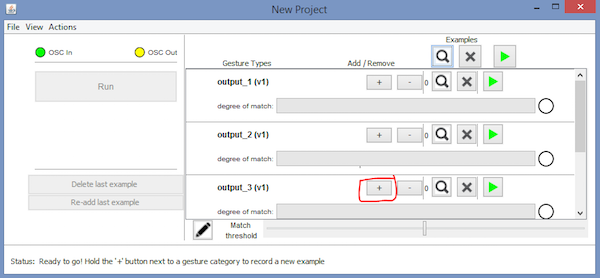Then hold the “+” button in front of the output_4 and say “left”.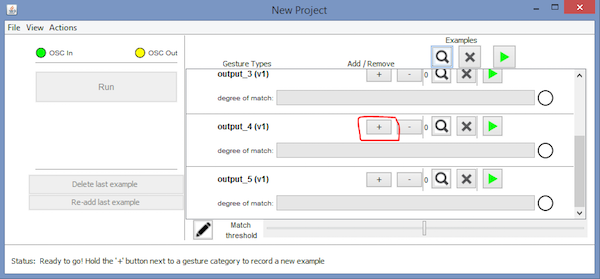Then hold the “+” button in front of output_5 and say “stop”.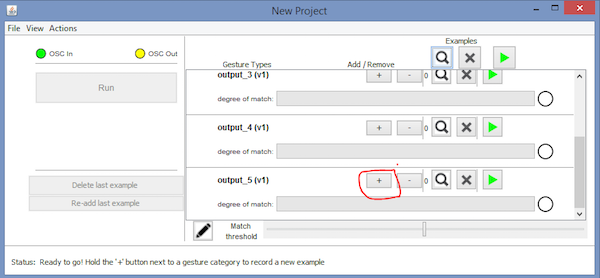After that, click on “Train”, then click “Run”. Make sure you have uploaded the Arduino code and that the processing sketch is running on the background. Now the motor should move based on your voice commands. Try testing with your voice and re-train if needed by repeating the above steps.

### How Does it Work?

In summary, there are three parts to this project: Wekinator, processing, and Arduino. Using machine learning, Wekinator is telling processing whether the voice it is listening to is a pre-trained voice command or not. Processing then reads that message and passes it to Arduino, then the Arduino decided whether to turn the motors clockwise/counterclockwise. All the communication that is happening between Wekinator and processing is through OSC (open sound control) protocol. The communication between processing and Arduino is done through serial.

### Arduino Code

``````#include <VSync.h>    //Including the library that will help us in receiving and sending the values from processing
Put the number of values to synchronize in the brackets */

/* The below variable will be synchronized in the processing
and it should be same on both sides. */
int output;

//Motor Pins
int EN_A = 11;
int IN1 = 9;
int IN2 = 8;
int IN3 = 7;
int IN4 = 6;
int EN_B = 10;

void setup()
{
/* Starting the serial communication because we are communicating with the
Processing through serial. The baudrate should be same as on the processing side. */
Serial.begin(19200);
//Initializing the motor pins as output
pinMode(EN_A, OUTPUT);
pinMode(IN1, OUTPUT);
pinMode(IN2, OUTPUT);
pinMode(IN3, OUTPUT);
pinMode(IN4, OUTPUT);
pinMode(EN_B, OUTPUT);

digitalWrite(EN_A, HIGH);
digitalWrite(EN_B, HIGH);

// Synchronizing the variable with the processing. The variable must be int type.
}

void loop()
{
// Receiving the output from the processing.

// Matching the received output to light up led's
if (output == 1)
{
//Forward
digitalWrite(IN1, LOW);
digitalWrite(IN2, HIGH);
digitalWrite(IN3, LOW);
digitalWrite(IN4, HIGH);
}
else if (output == 2)
{
//Backward
digitalWrite(IN1, HIGH);
digitalWrite(IN2, LOW);
digitalWrite(IN3, HIGH);
digitalWrite(IN4, HIGH);
}
else if (output == 3)
{
//Right
digitalWrite(IN1, LOW);
digitalWrite(IN2, HIGH);
digitalWrite(IN3, LOW);
digitalWrite(IN4, LOW);
}
else if (output == 4)
{
//Left
digitalWrite(IN1, LOW);
digitalWrite(IN2, LOW);
digitalWrite(IN3, HIGH);
digitalWrite(IN4, LOW);
}
else if (output == 5)
{
//Stop
digitalWrite(IN1, LOW);
digitalWrite(IN2, LOW);
digitalWrite(IN3, LOW);
digitalWrite(IN4, LOW);
}
}``````

### Processing Code

``````import vsync.*; // Importing the library that will help us in sending and receiving the values from the Arduino
import processing.serial.*;  // Importing the serial library

// Below libraries will connect and send, receive the values from wekinator
import oscP5.*;
import netP5.*;

// Creating the instances
OscP5 oscP5;
ValueSender sender;

// This variable will be syncronized with the Arduino and it should be same on the Arduino side.
public int output;

void setup()
{
// Starting the serial communication, the baudrate and the com port should be same as on the Arduino side.
Serial serial = new Serial(this, "COM10", 19200);
sender = new ValueSender(this, serial);

// Synchronizing the variable as on the Arduino side.
sender.observe("output");

// Starting the communication with wekinator. listen on port 12000, return messages on port 6448
oscP5 = new OscP5(this, 12000);
}

//This is called automatically when OSC message is received
void oscEvent(OscMessage theOscMessage) {
{
output = 1;
}
{
output = 2;
}
{
output = 3;
}
{
output = 4;
}
{
output = 5;
}
else
{

}
}

void draw()
{
//  Nothing to be drawn for this example
}``````